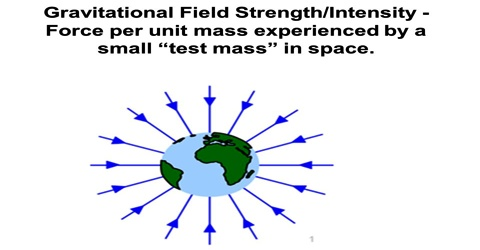Physics

# Gravitational Strength or IntensityGravitational Strength or Intensity

Intensity is not same in all points of the gravitational field. In order to determine the intensity or strength at any point in the gravitational field a unit mass is considered to be present there. Force experienced by the body of unit mass is the measure of gravitational field intensity. Gravitation is the weakest force among the four fundamental forces in nature. Gravitation played an important role in initiating the birth of stars, planets etc. and controlling the entire structure of the universe.

So, if force F acts on a body of mass m at a point in the gravitational field, then intensity or strength of gravitational field at that point is given by,

E = F/m … … … (1)

Intensity has both magnitude and direction. Direction of the intensity is the direction of the gravitational field.

Force experimented by a unit mass placed at a point in the gravitational field is called the intensity at the gravitational field at that point.

In S. I. system unit of intensity is Nkg-1.

The SI unit of gravitational field intensity is Newton per kilogram or metre per squared second and in CGS system the unit of gravitational field intensity is dyne per gram or centimetre per squared second. The gravitational field intensity is a vector quantity.